Previous: cyclone-Euler case Up: Case structure Next: Initial and boundary condition
This is an automatically generated documentation by LaTeX2HTML utility. In case of any issue, please, contact us at info@cfdsupport.com.

## Initial and boundary condition for twoPhaseEulerFoam

• The directory 0.euler contains the definition of initial and boundary condition for each variable.
• The list of all variables contains Table.
• Basic setup of initial and boundary condition:
• alphat.* – Set as calculated; At walls for continuous phase a standard wall function is applied.
• alpha.particles – Initial value: uniform 0; Inlet value: uniform 0.05.
• alpha.water – Initial value: uniform 1; Inlet value: uniform 0.95.
• epsilon.water – Initial and inlet value: uniform 3.144.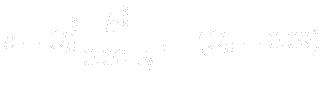• k.water – Initial and inlet value: uniform 0.375.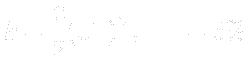• nut.* – Set as calculated; At walls for continuous phase a standard wall function is applied.
• p – Set as calculated; Computed from p_rgh.
• p_rgh – Pressure value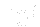is set at the outlet.
• Theta.particles – Small value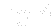is set at the inlet.
• T.* – Initial and inlet temperature is set to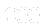K.
• U.*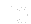m/s is set at the inlet (direction perpendicular to the inlet face).

Table: Initial and boundary conditions for twoPhaseEulerFoam.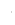0.euleralphat.particles <#37075#>alphat.wateralpha.particles <#37078#>alpha.waterepsilon.water <#37081#>k.waternut.particlesnut.waterp - static pressurep_rgh -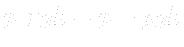Theta.particles - granular temperatureT.particles <#37092#>T.waterU.particles <#37095#>U.water